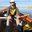Question-and-Answer Resource for the Building Energy Modeling Community
Get started with the Help page

# Energy Plus, calculate zone energy balance manuallyHi!

I have some doubt about how to calculate a zone's energy balance manually, using EnergyPlus output variables. Using a most simple model with only walls and no window, light, equipment, people, infiltration and a simple ac this works quite well (i.e. balance is close to zero), summing up these variables:

• Surface Inside Face Conduction Heat Transfer Rate,
• Zone Electric Equipment Total Heating Rate,
• People Sensible Heating Rate,
• Zone Lights Total Heating Rate,
• Zone Air System Sensible Cooling Rate,
• Zone Air System Sensible Heating Rate (of walls, ceiling and floor ),
• Zone Infiltration Sensible Heat Loss Energy,
• Zone Infiltration Sensible Heat Gain Energy.

Using a slightly more complicated model including a window I simply added the according variable for the window:

• Surface Window Heat Loss Rate,
• Surface Window Heat Gain Rate,

but the balance (i.e. sum) is far from being zero. Is there any point I am missing?

Best regards

Chris

EDIT EDIT EDIT

Hi! Thanks a lot everyone for the quick and wonderful replies. Here some more information about what I was doing as I didn't write it above.

The difference for the case I am looking gets up to 90 W / h for an about 35 m² room. What I was actually looking at is the annual sum of these values and expected it to be zero.

As far as I understand the EP manual the inside conduction should get quite close to what is actually transferred by a wall. At least in a case with more zones and an interior wall between them the both values of the two zones match quite closely. The according convective variables should give the heat transferred from the wall to the air, right?

The reason for the question is an analysis where I want to check through which surface most heat enter the zone and I fully agree that there could (should??) be a simple solution for this from the side of EP, which we have been discussing here as well. Cheers Chris

EDIT EDIT EDIT

edit retag close merge delete

How far from zero? The items you listed shouldn't sum exactly to zero because the HVAC controller almost never keeps the zone air exactly at setpoint. The net amount of energy that doesn't balance will cause the space temperature to slightly drift up or down in the next time-step.

1

And in the zone air balance heat equation, it is not the 'inside face conduction heat transfer' rate that represents the heat transmission through envelope, but those capturing the heat transfer between the surface and the air. But all in all, in my opinion getting a manual heat balance in EP is not a trivial task and it is a pity as it can be very helpful to understand the building performance. I had put a request to address this issue in the feedback site. https://energyplus.uservoice.com/foru...

Sort by » oldest newest most votedThey are not manual, but this set of output variables provides results that are useful for understanding what is driving heat transfer in the zone air balance.

• Zone Air Heat Balance Internal Convective Heat Gain Rate [W]
• Zone Air Heat Balance Surface Convection Rate [W]
• Zone Air Heat Balance Interzone Air Transfer Rate [W]
• Zone Air Heat Balance Outdoor Air Transfer Rate [W]
• Zone Air Heat Balance System Air Transfer Rate [W]
• Zone Air Heat Balance System Convective Heat Gain Rate [W]
• Zone Air Heat Balance Air Energy Storage Rate [W]
• Zone Air Heat Balance Deviation Rate [W]

This output variable is probably the simplest way to compare different opaque surfaces (of relatively lightweight contruction).

• Surface Average Face Conduction Heat Transfer Rate per Area [W/m2]
more